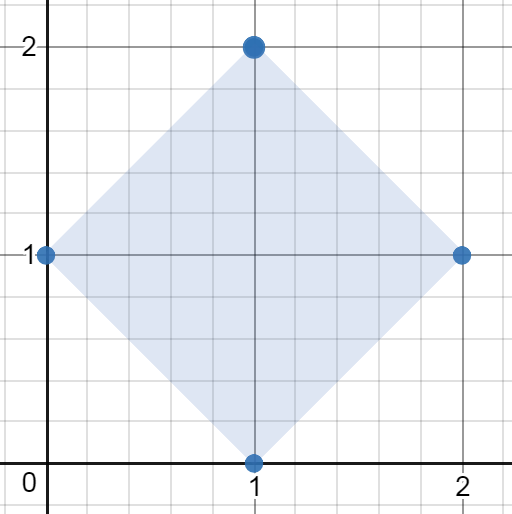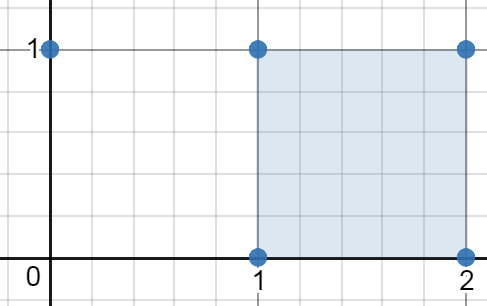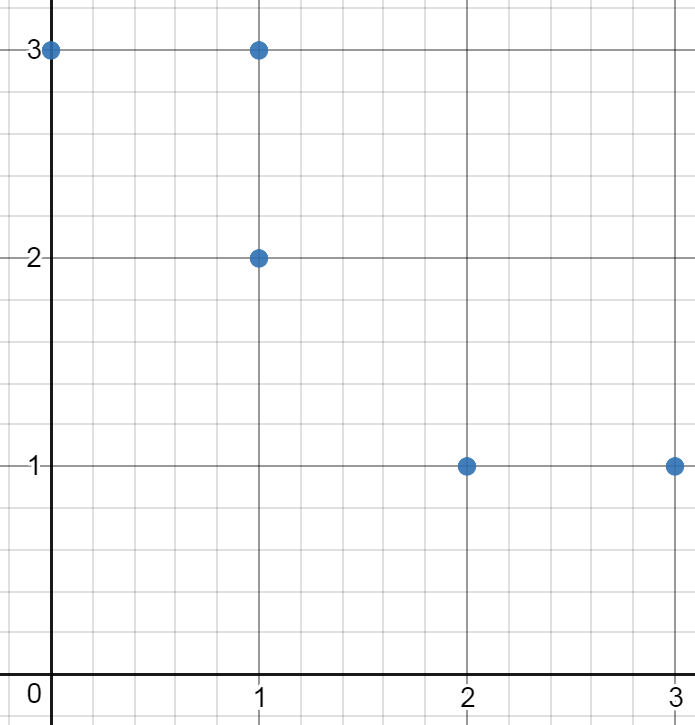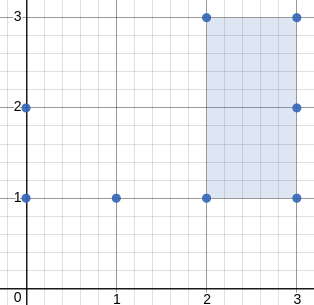##### Welcome to Subscribe On Youtube

Formatted question description: https://leetcode.ca/all/963.html

# 963. Minimum Area Rectangle II

Medium

## Description

Given a set of points in the xy-plane, determine the minimum area of any rectangle formed from these points, with sides not necessarily parallel to the x and y axes.

If there isn’t any rectangle, return 0.

Example 1:Input: [[1,2],[2,1],[1,0],[0,1]]

Output: 2.00000

Explanation: The minimum area rectangle occurs at [1,2],[2,1],[1,0],[0,1], with an area of 2.

Example 2:Input: [[0,1],[2,1],[1,1],[1,0],[2,0]]

Output: 1.00000

Explanation: The minimum area rectangle occurs at [1,0],[1,1],[2,1],[2,0], with an area of 1.

Example 3:Input: [[0,3],[1,2],[3,1],[1,3],[2,1]]

Output: 0

Explanation: There is no possible rectangle to form from these points.

Example 4:Input: [[3,1],[1,1],[0,1],[2,1],[3,3],[3,2],[0,2],[2,3]]

Output: 2.00000

Explanation: The minimum area rectangle occurs at [2,1],[2,3],[3,3],[3,1], with an area of 2.

Note:

1. 1 <= points.length <= 50
2. 0 <= points[i] <= 40000
3. 0 <= points[i] <= 40000
4. All points are distinct.
5. Answers within 10^-5 of the actual value will be accepted as correct.

## Solution

For each group of three points, if the three points form a right triangle, then find the right angle and try to find another point such that the four points can form a rectangle, and calculate the area. If a rectangle is found, update the minimum area. If no rectangle is found, return 0.

• class Solution {
public double minAreaFreeRect(int[][] points) {
if (points == null || points.length < 4 || points.length != 2)
return 0;
int length = points.length;
double minArea = Double.MAX_VALUE;
int end1 = length - 3, end2 = length - 2, end3 = length - 1;
for (int i = 0; i < end1; i++) {
int[] point1 = points[i];
for (int j = i + 1; j < end2; j++) {
int[] point2 = points[j];
for (int k = j + 1; k < end3; k++) {
int[] point3 = points[k];
if (isRightAngle(point1, point2, point3)) {
for (int m = k + 1; m < length; m++) {
int[] point4 = points[m];
double area = rectangleArea(point1, point2, point3, point4);
if (area > 0)
minArea = Math.min(minArea, area);
}
} else if (isRightAngle(point2, point3, point1)) {
for (int m = k + 1; m < length; m++) {
int[] point4 = points[m];
double area = rectangleArea(point2, point3, point1, point4);
if (area > 0)
minArea = Math.min(minArea, area);
}
} else if (isRightAngle(point3, point1, point2)) {
for (int m = k + 1; m < length; m++) {
int[] point4 = points[m];
double area = rectangleArea(point3, point1, point2, point4);
if (area > 0)
minArea = Math.min(minArea, area);
}
}
}
}
}
return minArea == Double.MAX_VALUE ? 0 : minArea;
}

public double rectangleArea(int[] point1, int[] point2, int[] point3, int[] point4) {
int x = point1 + point3 - point2, y = point1 + point3 - point2;
if (point4 == x && point4 == y) {
double side1 = Math.sqrt((point1 - point2) * (point1 - point2) + (point1 - point2) * (point1 - point2));
double side2 = Math.sqrt((point2 - point3) * (point2 - point3) + (point2 - point3) * (point2 - point3));
return side1 * side2;
} else
return 0;
}

public boolean isRightAngle(int[] point1, int[] point2, int[] point3) {
return (point1 - point2) * (point2 - point3) + (point1 - point2) * (point2 - point3) == 0;

}
}

• // OJ: https://leetcode.com/problems/minimum-area-rectangle-ii/
// Time: O(N^3)
// Space: O(N)
// Ref: https://leetcode.com/problems/minimum-area-rectangle-ii/solution/
class Solution {
double dist(vector<int> &a, vector<int> &b) {
return sqrt(pow(a - b, 2) + pow(a - b, 2));
}
public:
double minAreaFreeRect(vector<vector<int>>& A) {
int N = A.size();
set<vector<int>> s(begin(A), end(A));
double ans = DBL_MAX;
for (int i = 0; i < N; ++i) {
auto &p1 = A[i];
for (int j = 0; j < N; ++j) {
if (i == j) continue;
auto &p2 = A[j];
for (int k = j + 1; k < N; ++k) {
if (k == i) continue;
auto &p3 = A[k], p4 = vector<int>{ p2 + p3 - p1, p2 + p3 - p1 };
if (s.count(p4)) {
int dot = (p2 - p1) * (p3 - p1) + (p2 - p1) * (p3 - p1);
if (dot == 0) {
double area = dist(p1, p2) * dist(p1, p3);
ans = min(ans, area);
}
}
}
}
}
return ans == DBL_MAX ? 0 : ans;
}
};

• class Solution(object):
def minAreaFreeRect(self, points):
"""
:type points: List[List[int]]
:rtype: float
"""
N = len(points)
# (l^2, x#, y#) : [(0,1), (1,2)]
d = collections.defaultdict(list)
for i in range(N - 1):
pi = points[i]
for j in range(i + 1, N):
pj = points[j]
l = (pi - pj) ** 2 + (pi - pj) ** 2
x = (pi + pj) / 2.0
y = (pi + pj) / 2.0
d[(l, x, y)].append((i, j))
res = float("inf")
for l in d.values():
M = len(l)
for i in range(M - 1):
p0, p2 = points[l[i]], points[l[i]]
for j in range(i + 1, M):
p1, p3 = points[l[j]], points[l[j]]
d1 = math.sqrt((p0 - p1) ** 2 + (p0 - p1) ** 2)
d2 = math.sqrt((p1 - p2) ** 2 + (p1 - p2) ** 2)
area = d1 * d2
res = min(res, area)
return 0 if res == float('inf') else res